Ex 10.1

Chapter 10 Class 11 Conic Sections
Serial order wise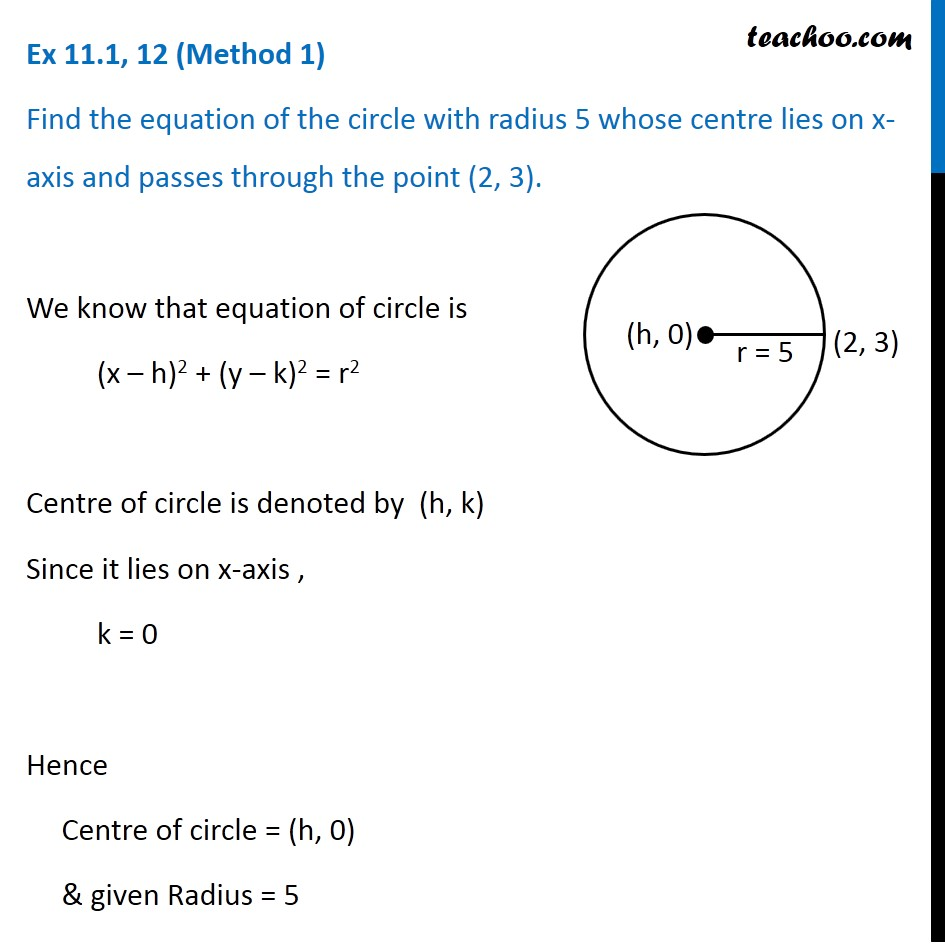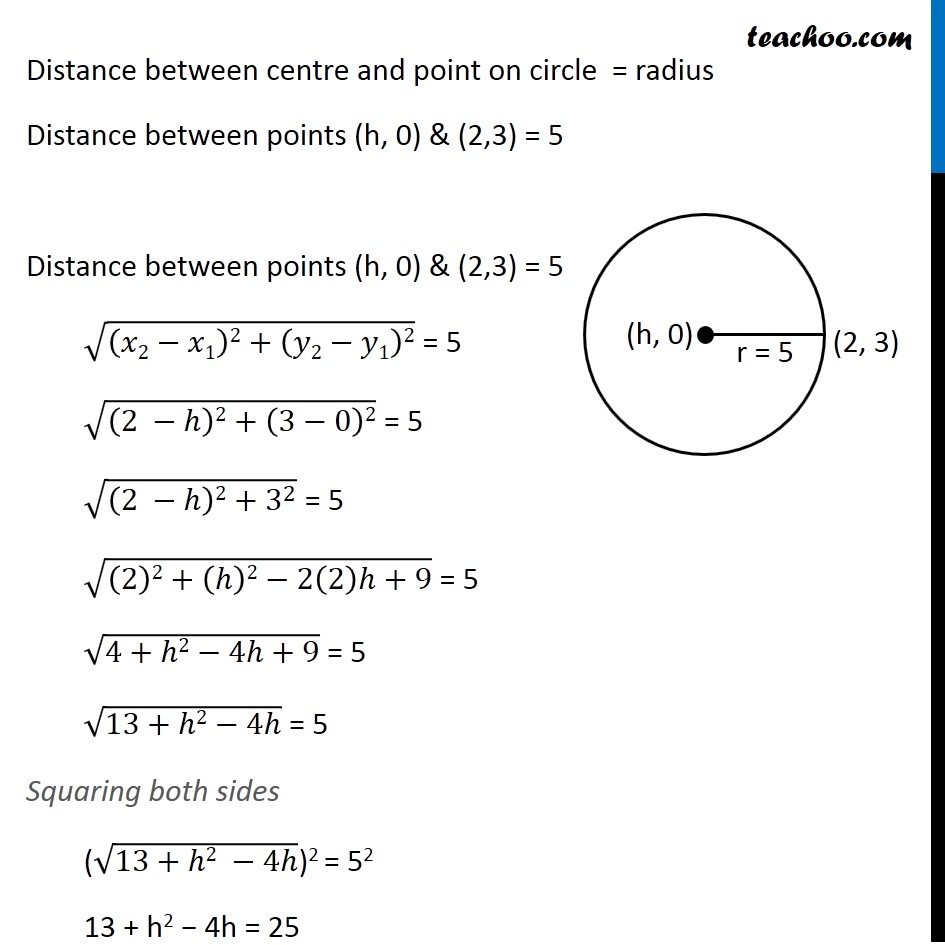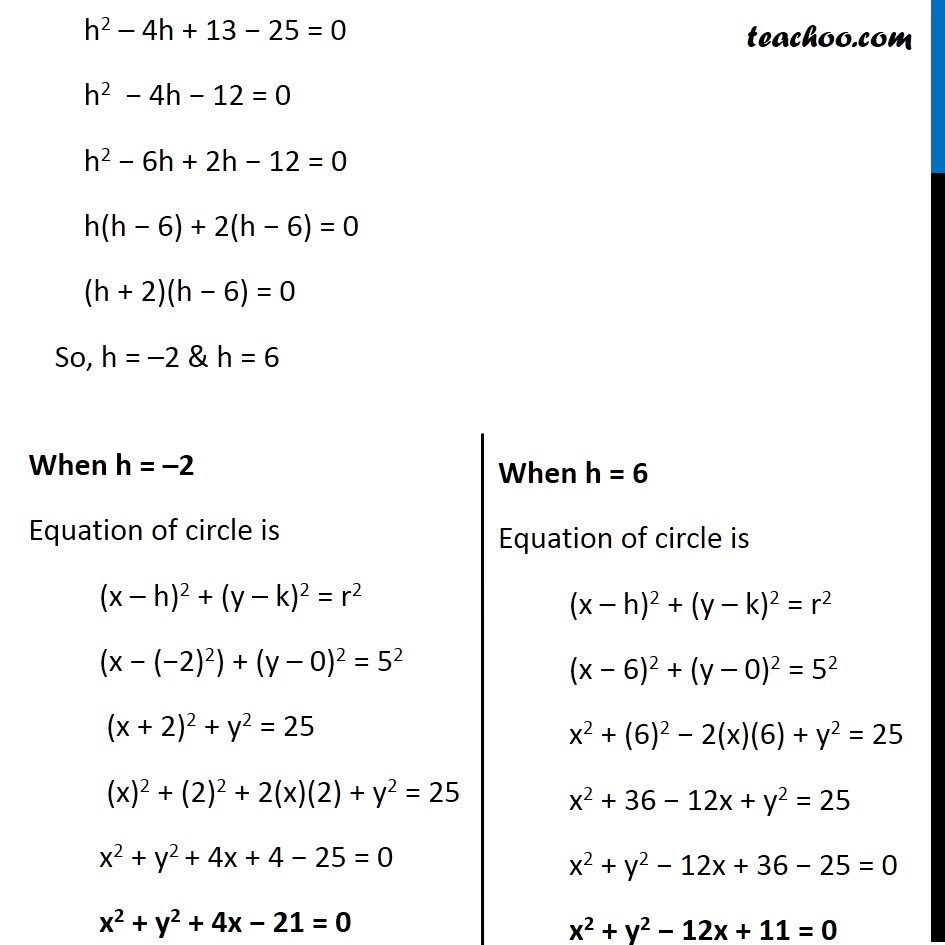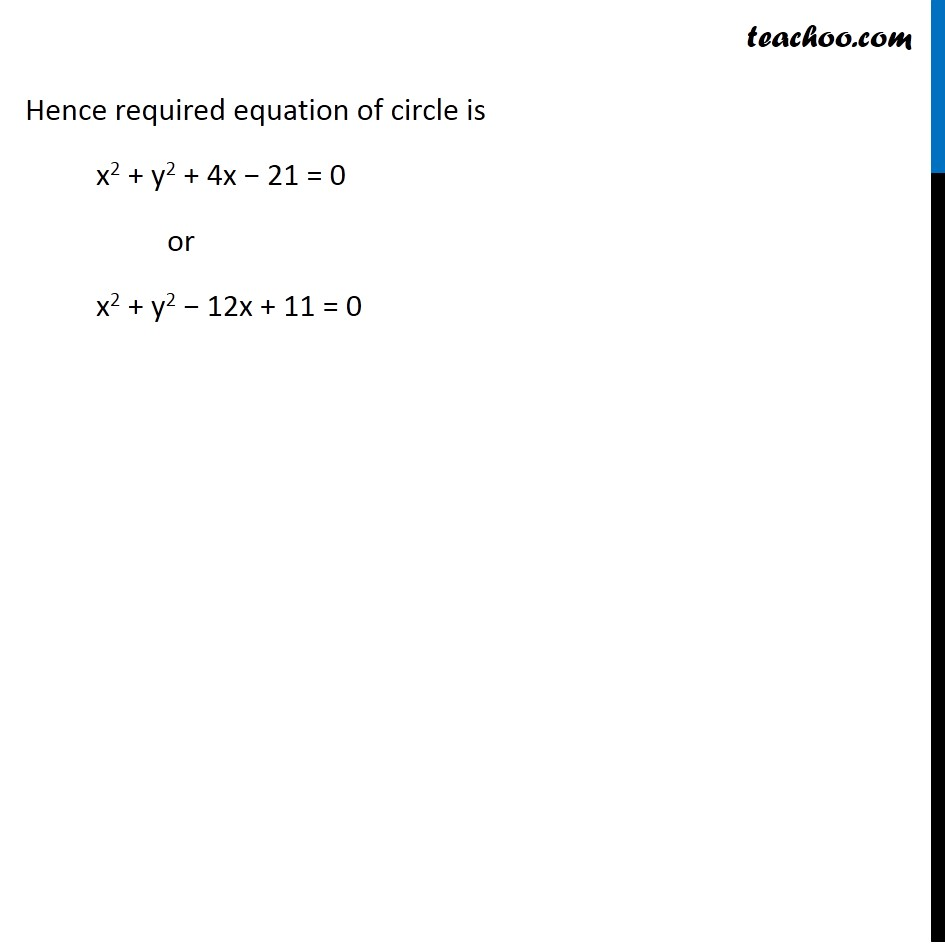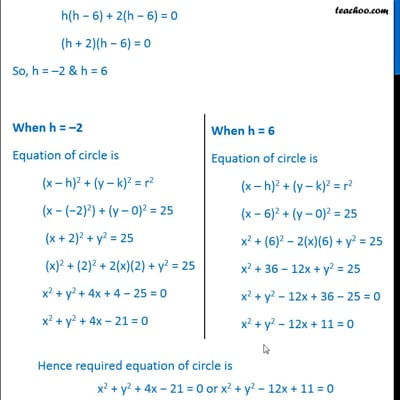This video is only available for Teachoo black users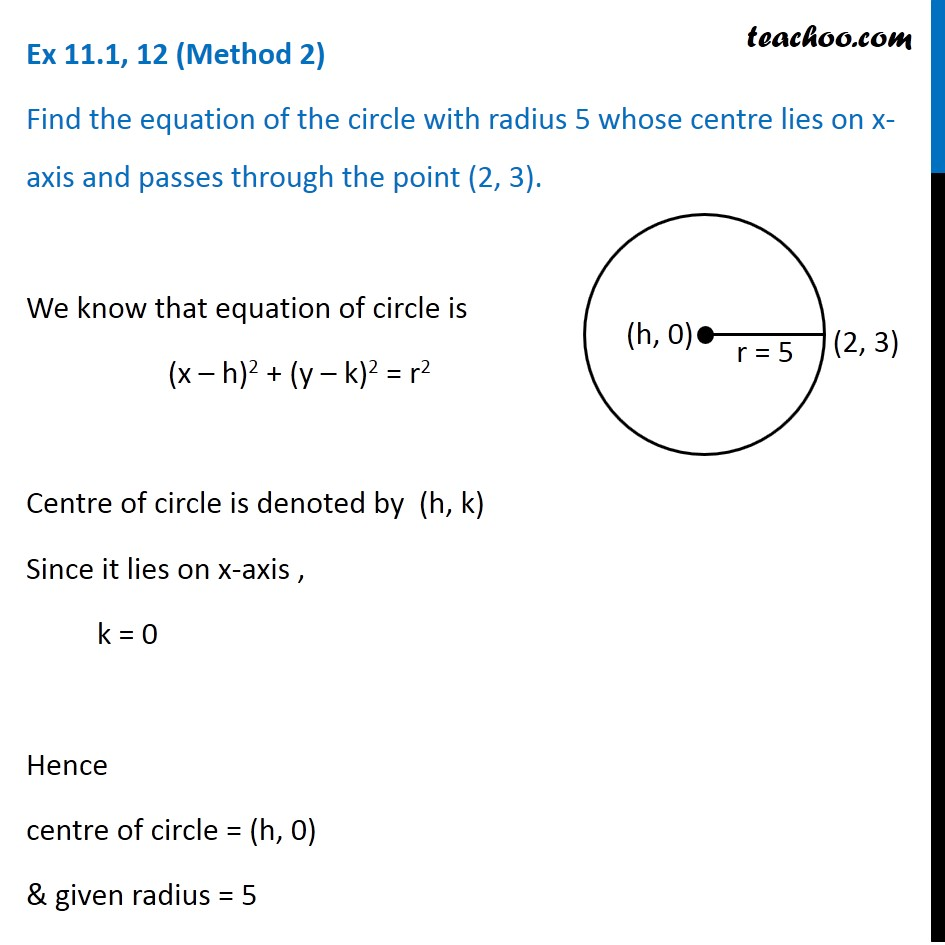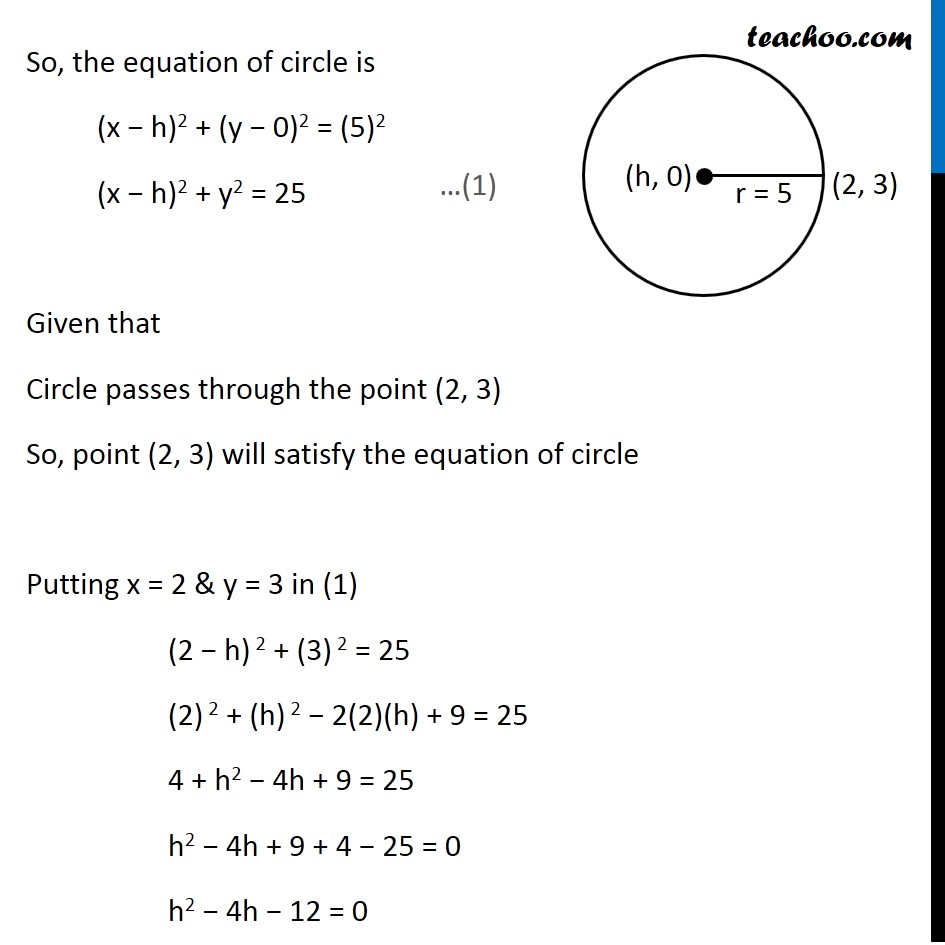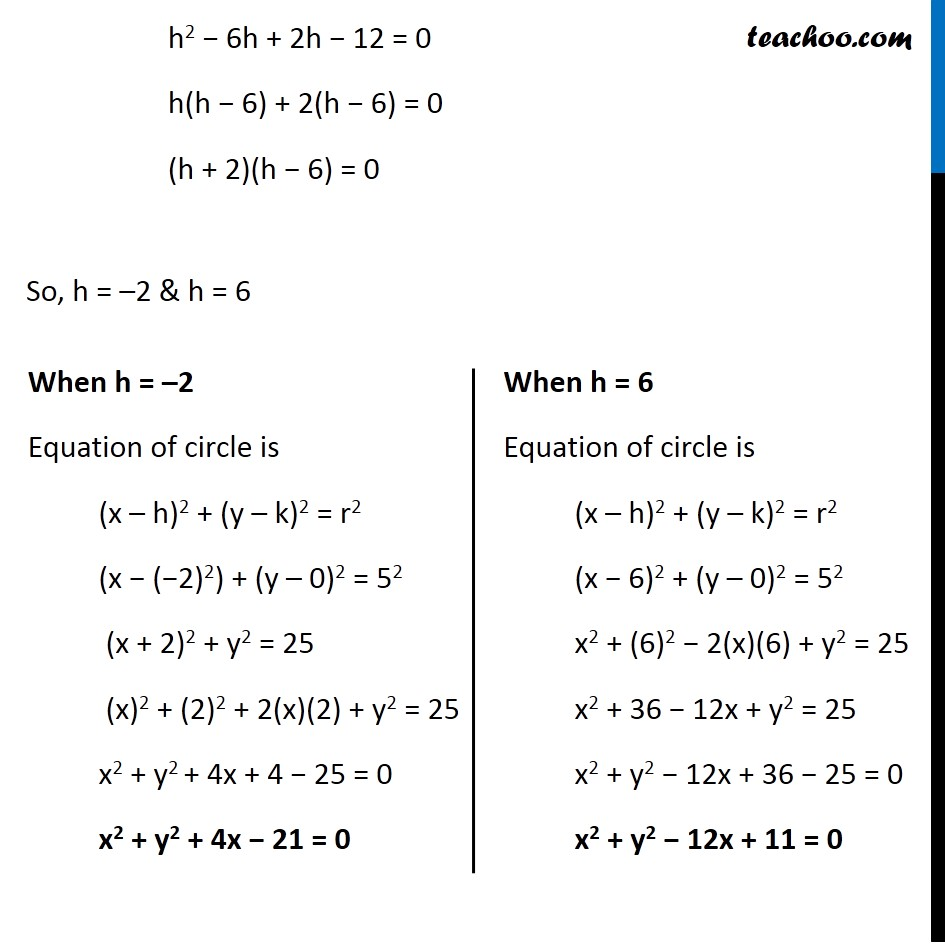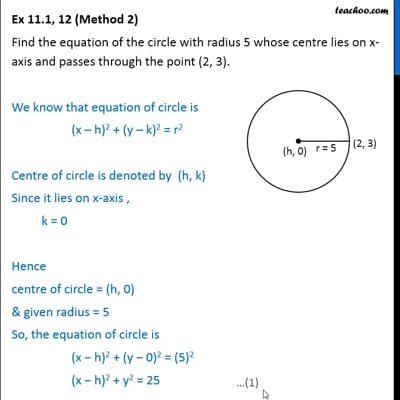This video is only available for Teachoo black users

Learn in your speed, with individual attention - Teachoo Maths 1-on-1 Class

### Transcript

Ex 10.1, 12 (Method 1) Find the equation of the circle with radius 5 whose centre lies on x-axis and passes through the point (2, 3). We know that equation of circle is (x – h)2 + (y – k)2 = r2 Centre of circle is denoted by (h, k) Since it lies on x-axis , k = 0 Hence Centre of circle = (h, 0) & given Radius = 5 Distance between centre and point on circle = radius Distance between points (h, 0) & (2,3) = 5 Distance between points (h, 0) & (2,3) = 5 √((𝑥2−𝑥1)2+(𝑦2−𝑦1)2) = 5 √((2 −ℎ)2+(3−0)2) = 5 √((2 −ℎ)2+3^2 ) = 5 √((2)2+(ℎ)2−2(2)ℎ+9) = 5 √(4+ℎ2−4ℎ+9) = 5 √(13+ℎ2−4ℎ) = 5 Squaring both sides (√(13+ℎ2 −4ℎ))2 = 52 13 + h2 − 4h = 25 h2 – 4h + 13 − 25 = 0 h2 − 4h − 12 = 0 h2 − 6h + 2h − 12 = 0 h(h − 6) + 2(h − 6) = 0 (h + 2)(h − 6) = 0 So, h = –2 & h = 6 When h = –2 Equation of circle is (x – h)2 + (y – k)2 = r2 (x − (−2)2) + (y – 0)2 = 52 (x + 2)2 + y2 = 25 (x)2 + (2)2 + 2(x)(2) + y2 = 25 x2 + y2 + 4x + 4 − 25 = 0 x2 + y2 + 4x − 21 = 0 When h = 6 Equation of circle is (x – h)2 + (y – k)2 = r2 (x − 6)2 + (y – 0)2 = 52 x2 + (6)2 − 2(x)(6) + y2 = 25 x2 + 36 − 12x + y2 = 25 x2 + y2 − 12x + 36 − 25 = 0 x2 + y2 − 12x + 11 = 0 Hence required equation of circle is x2 + y2 + 4x − 21 = 0 or x2 + y2 − 12x + 11 = 0 Ex 10.1, 12 (Method 2) Find the equation of the circle with radius 5 whose centre lies on x-axis and passes through the point (2, 3). We know that equation of circle is (x – h)2 + (y – k)2 = r2 Centre of circle is denoted by (h, k) Since it lies on x-axis , k = 0 Hence centre of circle = (h, 0) & given radius = 5 So, the equation of circle is (x − h)2 + (y − 0)2 = (5)2 (x − h)2 + y2 = 25 Given that Circle passes through the point (2, 3) So, point (2, 3) will satisfy the equation of circle Putting x = 2 & y = 3 in (1) (2 − h) 2 + (3) 2 = 25 (2) 2 + (h) 2 − 2(2)(h) + 9 = 25 4 + h2 − 4h + 9 = 25 h2 − 4h + 9 + 4 − 25 = 0 h2 − 4h − 12 = 0 h2 − 6h + 2h − 12 = 0 h(h − 6) + 2(h − 6) = 0 (h + 2)(h − 6) = 0 So, h = –2 & h = 6 When h = –2 Equation of circle is (x – h)2 + (y – k)2 = r2 (x − (−2)2) + (y – 0)2 = 52 (x + 2)2 + y2 = 25 (x)2 + (2)2 + 2(x)(2) + y2 = 25 x2 + y2 + 4x + 4 − 25 = 0 x2 + y2 + 4x − 21 = 0 When h = 6 Equation of circle is (x – h)2 + (y – k)2 = r2 (x − 6)2 + (y – 0)2 = 52 x2 + (6)2 − 2(x)(6) + y2 = 25 x2 + 36 − 12x + y2 = 25 x2 + y2 − 12x + 36 − 25 = 0 x2 + y2 − 12x + 11 = 0 Hence required equation of circle is x2 + y2 + 4x − 21 = 0 or x2 + y2 − 12x + 11 = 0